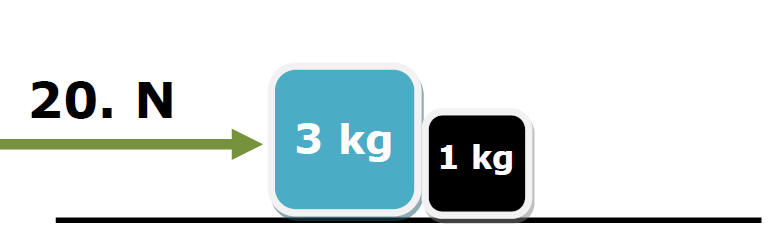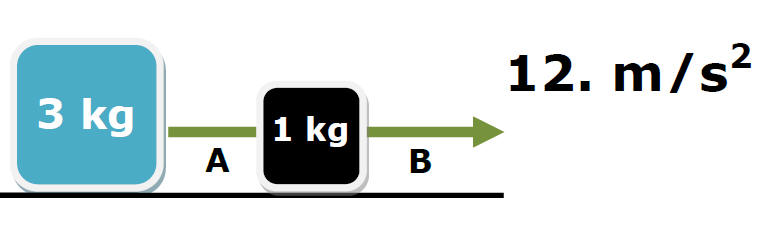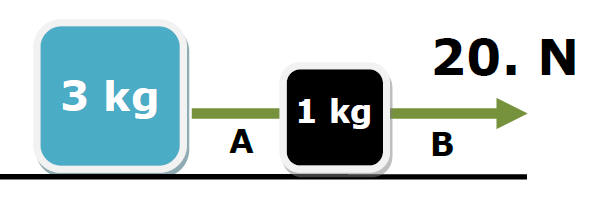﻿ HomeHomeEx 1)frictionless surfaceFind the force of the 3 kg box on the 1 kg box?   Both boxes a = 5 m/s2   Fnet = ma = 1kg(5 m/s2) = 5 NNet force on 3 kg box?   20 N - 4 N = 15 N   or Fnet = ma = 3 kg(5 m/s2) = 15 N Ex 2) frictionless surfaceNet force on 1 kg mass?   Tension B = 48 N Tension A = 36 NFnet on 1 kg =   12 N Ex 3)Find Rope Tension force A Fnet = ma 20. N = (4 kg)a a = 5 m/s2Net force on 1 kg?   F = ma = 5 ND©Tony Mangiacapre., St. Mary's H.S., Manhasset, NY All Rights Reserved [Home] Established 1995